# 前言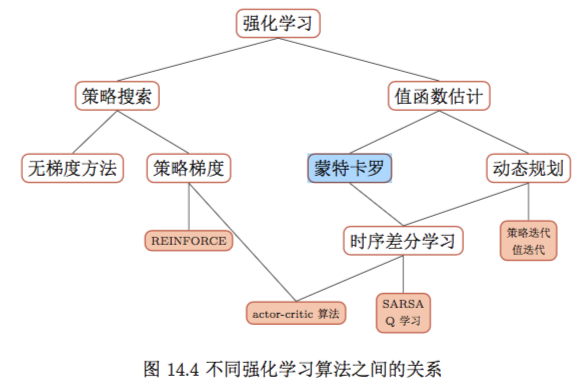# 概述

## 马尔科夫决策过程（MDP）

• S是一个有限状态集，就是你处于哪个状态啊
• A是说采取啥动作
• P 是状态转移概率矩阵：$P^a_{s->s′}$ ，就是你在s，采取a，那么你跳转到下一个状态的s’的转移概率
• R 是一个奖励函数，$R_s^a = E[R_{t+1}|S_t = s,A_t=a]$，注意，是个期望哈，这个“期望”的概念很重要
• $\gamma$是一个衰减因子: $\gamma\in$[0,1]，这个是为了让整个收益收敛。

$\color{blue}{画外音}$

## 收益（$G_t$）

$G_t = R_{t+1} + \gamma R_{t+2} + \gamma^2 R_{t+3} … = \sum_{k=0}^{\infty} \gamma^k R_{t+k+1}$

$\color{blue}{画外音}$

BUT，不过，但是，你注意到了么，他的视角不是游戏开头到游戏结束，他是基于某个状态的。我靠，你可能奇怪了，为毛呢？你干嘛从一个状态开始测算收益呢？恩，问的好！是因为，你丫在决策过程中的时候，你玩到半截了，你不是上帝视角，你深陷游戏当中，当局者正迷呢，“兄弟！求你就告诉我，我选a1好，还是选a2，还是选…”，好，我告诉你：你就选择，a之后，从a的那个状态开始，到最后的受益最大的那个a吧。冥冥之中，你就听到我的这个点拨，你就可以毅然决然地选择某个a，杀出重围，继续前行。其实，哪有什么冥冥，这个冥冥就是策略$\pi$啊，“理科男！信概率，别信命！”

## 价值（Value）

$v(s)= E[G_t|S_t = s]$

$\color{blue}{画外音}$

## 价值函数（Value Function）

\begin{align} & v(s)=E(G_t|S_t=s) \tag{1}\\ & =E(R_{t+1} + \gamma R_{t+2} + \gamma^2 R_{t+3} ... |S_t=s) \\ & =E(R_{t+1} + \gamma ( R_{t+2} + \gamma R_{t+3} ...) |S_t=s) \\ & =E(R_{t+1} + \gamma G_{t+1} |S_t=s) \\ & =E(R_{t+1} + \gamma v(s_{t+1}) |S_t=s) \\ \end{align}

$v(s) = E(R_{t+1} + \gamma * v(s_{t+1}) |S_t=s)$### 换个角度

（其实，不是为了换个啥角度，只是因为，我之前写了一大坨，不舍得删，所以，再让你“换个角度”，再看一遍。哈哈。不过，应该是有点用的，耐心，patient！）

#### 轨迹（τ）

$τ = s_0,a_0,s_1,r_1,a_1,··· ,s_{t−1},a_{t−1},s_t ,r_t$，我们来算算这个$τ$的概率：

#### 轨迹概率（$p(τ)$）

$p(τ) = p(s_0, a_0, s_1, a_1, · · · ) \\ = p(s_0) \prod_{t=0}^{T-1} \pi(a_t\|s_t) p(s_{t+1}\|s_t,a_t)$

#### 收益（$G_t$）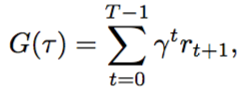$\gamma$是折现因子，在[0,1]之间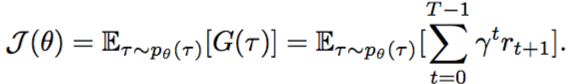# 基于模型[Model based]的策略求解

## 上帝视角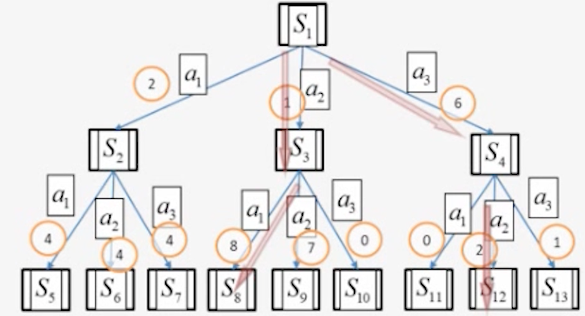## 贝尔曼方程

$v(s) = R_s + \gamma * \sum\limits_{s’\in S} P_{ss’} * v(s’)$

$\color{blue}{看个例子}$## 状态价值函数$v(s)$、行为价值函数 $q_\pi(s,a)$

$q_\pi(s,a) = E(G_t | S_t = s, A_t = a)$

$q_\pi(s,a) = E(R_{t+1} + \gamma q_\pi(S_{t+1},A_{t+1}) | S_t = s, A_t = a)$

$q_\pi(s,a) = R_{t+1} + \gamma \sum \limits_{s’\in S} P^a_{ss’} v_\pi(s’)$$v_\pi(s) = \sum \limits_{a\in A} \pi(a|s) q_\pi(s,a)$

$v_\pi(s) = \sum \limits_{a\in A} \pi(a|s) \left( R_s^a + \gamma \sum\limits_{s’\in S} P^a_{ss’} v_\pi(s’) \right)$

$q_\pi(s,a) = R_s^a + \gamma \sum \limits_{s\in S} P^a_{ss’} \sum\limits_{a’\in A} \pi(a’|s’) q_\pi(s’,a’)$

## 最优状态价值函数、最优行为价值函数

$v_*(s) = \max\limits_\pi v_\pi(s)$

$q_*(s,a) = \max\limits_\pi q_\pi(s,a)$

\pi_*(a\|s) = \left\{ \begin{align} \label{最优策略确定方法} 1 & \text{ 如果 } \arg\max\limits_{a \in A} q_\*(s,a) \\ 0 & \text{ 其他情况} \end{align} \right.

$v_\pi(s) = \sum\limits_{a\in A} \pi(a|s) q_\pi(s,a)$

ok，那我把最优策略$\pi_*$，带入到这个方程中，得到贝尔曼最优方程（原来就是把最优策略$\pi_*$带入进去就得到了啊，我之前还发愁怎么推导贝尔曼最优方程呢）

$v_\pi^*(s) = \sum\limits_{a\in A} \pi^*(a|s) q_\pi^*(s,a)$

$q_\pi^*(s,a) = R_s^a + \gamma \sum\limits_{s\in S} P^a_{ss’} v_*(s’)$

$v_\pi^*(s) = \max\limits_a q_\pi^*(s,a)$ ===> 注意哈：这个前提是采用了上面的最优策略确定方法才可以推出

$v_*(s) = \max \limits_a ( R_s^a + \gamma \sum\limits_{s\in S} P^a_{ss’} v_*(s’))$

$q_*(s,a) = R_s^a + \gamma \sum\limits_{s\in S} P^a_{ss’} \max\limits_{a’} q_*(s’,a’)$

## 策略迭代

### 例子$V_\pi(s) = E_\pi[G_t|S_t=s]$

$V_\pi(s) = E_\pi[R_{t+1}+\gamma G_{t+1}|S_t=s]$

$V_\pi(s) = E_\pi[R_{t+1}+\gamma V(S_{t+1})|S_t=s]$

$V_\pi(s) = \sum_a\pi(a|s)\sum_{s’}P(s’|s,a)(R(s,a,s′)+γV_\pi(s′))$

$V_{k+1}(s) = \sum_a\pi(a|s)\sum_{s’,r}P(s’,r|s,a)(r+γV_k(s′))$

$\color{blue}{算一算v([1,1]),k=1}$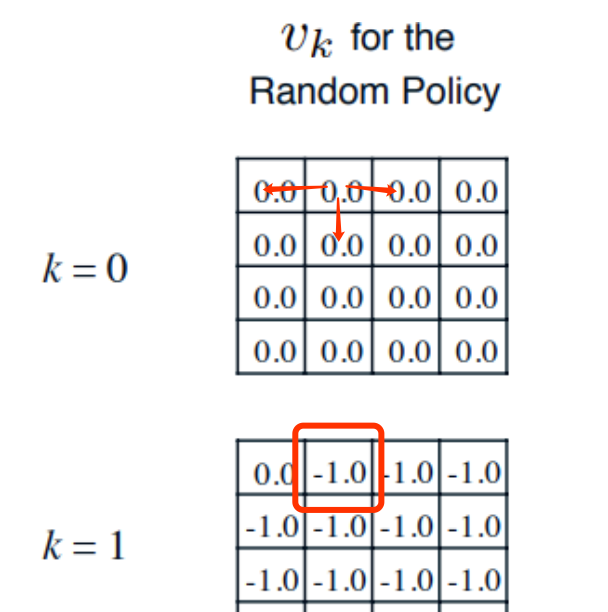$V_{k+1}(s) = \sum_a\pi(a|s)\sum_{s’,r}P(s’,r|s,a)(r+\gamma V_k(s′))$

$V_{k+1}(s) = \sum_a 0.25 (r+ V_k(s′))$

$v[1,1]= 0.25(-1+0) + 0.25(-1+0) + 0.25(-1+0) + 0.25(-1+0) = -1$

$\color{blue}{再算一算状态v([1,1]), k=2}$

k2=2时刻，第一行第二列的1.7怎么来的呢？$v[1,1]= 0.25(-1+0) + 0.25(-1-1) + 0.25(-1-1) + 0.25(-1-1) = -1.75$

$\color{red}{策略迭代算法}$ - 引自周志华老师的《西瓜书》和一个博客• 算法分两部分，一个是评估，一个是迭代
• 评估的停止条件是收敛，周志华老师那个条件是$V=V’$，另外一个的终止条件是$\Delta<\theta$，其实意思都是收敛。所以，叶强的《强化学习》的第3.2节用k=2的图来说明策略改进是非常让人误导的，应该是这个$v(s)$所有的值收敛后，才可以做策略迭代。具体是多少，我也不知道，k=？的时候。
• 一旦基于当前这个策略$\pi$，你一遍遍的迭代着算，$v(s)$（注意，是对每个状态s）都稳定了，你才从这个稳定的转改值里面，用贪心策略，为每一个s，找一个最优的动作。
• 啥叫“最优”，又得用贝尔曼方程算一遍，就是里面说的$\pi’(s)=argmax_{a\in A} Q(s,a)$，就是算一下每个动作的$Q(s,a)$值，最大的那个，就是我要的动作a。
• 那如果有多个a的$Q(s,a)$值都一样，我理解，就都留着，只不过，每个人的概率均等，也就是$p(a|s)$为1/|备选a|。

## 价值迭代

Q-learning和策略迭代区别？

Q-learning是

# 没有模型[Model Free]情况下的策略学习

## 蒙特卡洛方法

$G_t=r_{t+1} + \gamma r_{t+2} + \gamma^2 r_{t+3} + … + \gamma^{T-1}r_T$

$v(s)=E(G_t)$

\begin{align} &\mu_k = \frac{1}{k} \sum_{j=1}^k x_j \\ & = \frac{1}{k} (x_k + \sum_{j=1}^{k-1} x_j) \\ & = \frac{1}{k} (x_k + (k-1) \mu_{k-1}) \\ & = \mu_{k-1} + \frac{1}{k}(x_k - \mu_{k-1}) \\ \end{align}

• 新的均值（你也可以理解成为期望值），等于上一次迭代的均值，加上一个，新的值和上一次均值的差的1/k
• 注意，我们要求的就是均值，这个式子相当于不断的在修正这个均值，依据就是新的得到探索的值$x_k$，换句话，也就是说，你的每一次探索$x_k$，都是在帮你在修正均值。

\begin{align} &v(s_t) = v(s_t) + \frac{1}{N(s_t)} (G_t - v(s_t)) \\ & = v(s_t) + \alpha (G_t - v(s_t)) \\ \end{align}

$\alpha = \frac{1}{N(s_t)}$，这个是假设$N(s_t)$，这个$\alpha$就被认为是一个很小很小的常数了，我不知道，这么搞合理不，不过貌似都这么假设。

## 时序差分强化学习

\begin{align} & v(s_t) = v(s_t) + \alpha (G_t - v(s_t)) \\ & = v(s_t) + \alpha (r_{t+1} + v(s_{t+1}) - v(s_t)) \\ \end{align}

\begin{align} & v_\pi(s) = E_\pi( G_t \| S_t = s) \\ & = E_\pi[ \sum_{k=0}^\infty \gamma^k R_{t+k+1} \| S_t = s] \\ & = E_\pi[ R_{t+1} + \gamma \sum_{k=0}^\infty \gamma^k R_{t+k+2} \| S_t = s] \\ & = E_\pi[ R_{t+1} + \gamma v_\pi(S_{t+1}) \| S_t = s] \\ \end{align}

$$\color{blue}{V(S_t) \leftarrow V(S_t) + \alpha (R_{t+1} + \gamma V(S_{t+1}) - V(S_t))}$$

• 比蒙特卡洛方法好多了，那个是把整个$\tau$跑完一遍，得到一个$G_t$，然后再平均，去算某个状态的$v(s)$
• 而我TD可以每步得个分，就可以修正$v(s)$，对，是修正，不是平均，递归修正

## 不基于模型的策略改进

$\pi'(s)= \arg\max\limits_{a\in A}(R_s^a + P^a_{ss'}V(s'))$

\pi(a\|s) = \left\{ \begin{aligned} & \frac{\epsilon}{m} + 1 - \epsilon & 如果 a^*= \arg\max\limits_{a\in A} Q(s,a) \\ & \frac{\epsilon}{m} & 其他情况\\ \end{aligned} \right.

## 参考资料

#### 一些概念

• 策略迭代（Policy Iteration）
• 值迭代 （Value Iteration）
• 基于模型（Model based）
• 模型无关（Model free）
• 探索与开发（Exploreation-Exploitation）
• 马尔可夫过程（Markov Process）：具备马尔科夫性质的序列$p(s_{t+1}|s_t, … , s_0) = p(s_{t+1}|s_t)$
• 马尔科夫决策过程（MDP - Markov Decision Process）:在马尔可夫过程中 加入一个额外的变量:动作 a,$p(s_{t+1}|s_t, a_t, · · · , s_0, a_0) = p(s_{t+1}|s_t, a_t)$
• 确定性策略(Deterministic Policy)：就是张确定的s->a的确定表，当前s，做了某个a，去哪个s’
• 随机性策略(Stochastic Policy):是个概率，s->a->s’，这个s’不是确定的，是个归一化概率
• 轨迹(Trajectory)
• 值函数（Value Function）
• 状态值函数（V(s)） - V函数
• 状态-动作值函数（Q(s,a)）- Q函数
• 动态规划算法
• 策略评估(policy evaluation)
• 策略改进(policy improvement)
• 蒙特卡罗方法
• 同策略(on policy)
• 异策略(off policy)
• 时序差分学习(temporal-difference learning) - TD
• 贝尔曼方程
• 贝尔曼最优方程
• ε-贪心法
• SARSA算法（State Action Reward State Action，SARSA）
• Q学习(Q-Learning)算法
• 深度Q网络(deep Q-networks，DQN)
• 经验回放(experience replay)
• 目标网络冻结(freezing target networks)